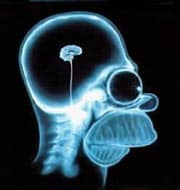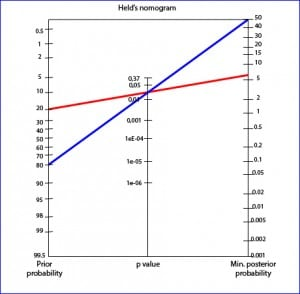# Science without sense…double nonsense

## Worshipped, but misunderstood

This post is also available in: Spanish

Statistics wears most of us who call ourselves “clinicians” out. The knowledge on the subject acquired during our formative years has long lived in the foggy world of oblivion. We vaguely remember terms such as probability distribution, hypothesis contrast, analysis of variance, regression … It is for this reason that we are always a bit apprehensive when we come to the methods section of scientific articles, in which all these techniques are detailed that, although they are known to us, we do not know with enough depth to correctly interpret their results.

Fortunately, Providence has given us a lifebelt: our beloved and worshipped p. Who has not felt lost with a cumbersome description of mathematical methods to finally breathe a sigh of relieve when finding the value of p? Especially if the p is small and has many zeros.

The problem with p is that, although it is unanimously worshipped, it is also mostly misunderstood. Its value is, very often, misinterpreted. And this is so because many of us harbor misconceptions about what the p-value really means.

Let’s try to clarify it.

Whenever we want to know something about a variable, the effect of an exposure, the comparison of two treatments, etc., we will face the ubiquity of random: it is everywhere and we can never get rid of it, although we can try to limit it and, of course, try to measure its effect.

Let’s give an example to understand it better. Suppose we are doing a clinical trial to compare the effect of two diets, A and B, on weight gain in two groups of participants. Simplifying, the trial will have one of three outcomes: those of diet A gain more weight, those of diet B gain more weight, both groups gain equal weight (there could even be a fourth: both groups lose weight). In any case, we will always obtain a different result, just by chance (even if the two diets are the same).

Imagine that those in diet A put on 2 kg and those in diet B, 3 kg. Is it more fattening the effect of diet B or is the difference due to chance (chosen samples, biological variability, inaccuracy of measurements, etc.)? This is where our hypothesis contrast comes in.

When we are going to do the test, we start from the hypothesis of equality, of no difference in effect (the two diets induce the same increment of weight). This is what we call the null hypothesis (H0) that, I repeat it to keep it clear, we assume that it is the real one. If the variable we are measuring follows a known probability distribution (normal, chi-square, Student’s t, etc.), we can calculate the probability of presenting each of the values of the distribution. In other words, we can calculate the probability of obtaining a result as different from equality as we have obtained, always under the assumption of H0.

That is the p-value: the probability that the difference in the result observed is due to chance. By agreement, if that probability is less than 5% (0.05) it will seem unlikely that the difference is due to chance and we will reject H0, the equality hypothesis, accepting the alternative hypothesis (Ha) that, in this example, will say that one diet better than the other. On the other hand, if the probability is greater than 5%, we will not feel confident enough to affirm that the difference is not due to chance, so we DO NOT reject H0 and we keep with the hypothesis of equal effects: the two diets are similar.

Keep in mind that we always move in the realm of probability. If p is less than 0.05 (statistically significant), we will reject H0, but always with a probability of committing a type 1 error: take for granted an effect that, in reality, does not exist (a false positive). On the other hand, if p is greater than 0.05, we keep with H0 and we say that there is no difference in effect, but always with a probability of committing a type 2 error: not detecting an effect that actually exists (false negative).

We can see, therefore, that the value of p is somewhat simple from the conceptual point of view. However, there are a number of common errors about what p-value represents or does not represent. Let’s try to clarify them.

It is false that a p-value less than 0.05 means that the null hypothesis is false and a p-value greater than 0.05 that the null hypothesis is true. As we have already mentioned, the approach is always probabilistic. The p <0.05 only means that, by agreement, it is unlikely that H0 is true, so we reject it, although always with a small probability of being wrong. On the other hand, if p> 0.05, it is also not guaranteed that H0 is true, since there may be a real effect that the study does not have sufficient power to detect.

At this point we must emphasize one fact: the null hypothesis is only falsifiable. This means that we can only reject it (with which we keep with Ha, with a probability of error), but we can never affirm that it is true. If p> 0.05 we cannot reject it, so we will remain in the initial assumption of equality of effect, which we cannot demonstrate in a positive way.

It is false that p-value is related to the reliability of the study. We can think that the conclusions of the study will be more reliable the lower the value of p, but it is not true either. Actually, the p-value is the probability of obtaining a similar value by chance if we repeat the experiment in the same conditions and it not only depends on whether the effect we want to demonstrate exists or not. There are other factors that can influence the magnitude of the p-value: the sample size, the effect size, the variance of the measured variable, the probability distribution used, etc.

It is false that p-value indicates the relevance of the result. As we have already repeated several times, p-value is only the probability that the difference observed is due to chance. A statistically significant difference does not necessarily have to be clinically relevant. Clinical relevance is established by the researcher and it is possible to find results with a very small p that are not relevant from the clinical point of view and vice versa, insignificant values that are clinically relevant.

It is false that p-value represents the probability that the null hypothesis is true. This belief is why, sometimes, we look for the exact value of p and do not settle for knowing only if it is greater or less than 0.05. The fault of this error of concept is a misinterpretation of conditional probability. We are interested in knowing what is the probability that H0 is true once we have obtained some results with our test. Mathematically expressed, we want to know P (H0 | results). However, the value of p gives us the probability of obtaining our results under the assumption that the null hypothesis is true, that is, P (results | H0).

Therefore, if we interpret that the probability that H0 is true in view of our results (P (H0 | results)) is equal to the value of p (P (results | H0)) we will be falling into an inverse fallacy or transposition of conditionals fallacy.

In fact, the probability that H0 is true does not depend only on the results of the study, but is also influenced by the previous probability that was estimated before the study, which is a measure of the subjective belief that reflects its plausibility, generally based on previous studies and knowledge. Let’s think we want to contrast an effect that we believe is very unlikely to be true. We will value with caution a p-value <0.05, even being significant. On the contrary, if we are convinced that the effect exists, will be settle for with little demands of p-value.

In summary, to calculate the probability that the effect is real we must calibrate the p-value with the value of the baseline probability of H0, which will be assigned by the researcher or by previously available data. There are mathematical methods to calculate this probability based on its baseline probability and the p-value, but the simplest way is to use a graphical tool, the Held’s nomogram, which you can see in the figure.To use the Held’s nomogram we just have to draw a line from the previous H0 probability that we consider to the p-value and extend it to see what posterior probability value we reach. As an example, we have represented a study with a p-value = 0.03 in which we believe that the probability of H0 is 20% (we believe there is 80% that the effect is real). If we extend the line it will tell us that the minimum probability of H0 is 6%: there is a 94% probability that the effect is real. On the other hand, think of another study with the same p-value but in which we think that the probability of the effect is lower, for example, of 20% (the probability of H0 is 80%). For the same value of p, the minimum posterior probability of H0 is 50%, then there is 50% that the effect is real. As we can see, the posterior probability changes according to the previous probability.

And here we will end for today. We have seen how p-value only gives us an idea of the role that chance may have had in our results and that, in addition, may depend on other factors, perhaps the most important the sample size. The conclusion is that, in many cases, the p-value is a parameter that allows to assess in a very limited way the relevance of the results of a study. To do it better, it is preferable to resort to the use of confidence intervals, which will allow us to assess clinical relevance and statistical significance. But that is another story…

This site uses Akismet to reduce spam. Learn how your comment data is processed.